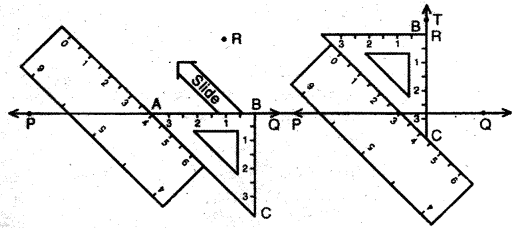# Class 6 Maths NCERT Solutions for Chapter 14 Practical Geometry Ex – 14.4

## Practical Geometry

Question 1.
Draw any line segment$\overline { AB }$,. Mark any point M on it. Through M, draw a perpendicular to$\overline { AB }$,. (use ruler and compasses)

Solution:
Steps of Construction:1. Draw a line segment AB, and mark any point M on it.
2. With centre M and any radius, cut off MX and MY of equal lengths on both sides of M.
3. With centre X and any radius > MX, draw an arc.
4. With centre Y and the same radius draw another arc, cutting the previously drawn arc at P
5. Join MP
Then, the segment PM so obtained is the required perpendicular.

Question 2.
Draw any line segment$\overline { PQ }$,. Take any point R not on it. Through R, draw a perpendicular to$\overline { PQ }$,. (use ruler and set-square)

Solution:
Steps of Construction:

1. Let PQ be the line and R is any point not lying on PQ.
2. Place the set-square so that the base AB of the set-square lies exactly on the line PQ.
3. Hold the set-square fixed and place a ruler so that its edge position lies along the side AC of the set-square.
4. Holding the ruler fixed, slide the set-square along the ruler till the point R coincides with the point B of the set-square.
5. Keeping the set-square fixed in this position, draw a line RT along the edge BC of the set-square through R.Thus, RT is the required perpendicular line to the line PQ passing through R.

Question 3.

Draw a line l and a point X on it. Through X, draw a line segment$\overline { XY }$ perpendicular to l. Now draw a perpendicular to XY at Y. (use ruler and compasses)

Solution:
Steps of Construction:1. Draw a line l and mark any point X on it. ,
2. With centre X and any radius, cut off XA = XB on both sides of X.
3. With centre A and any radius > XA, draw an arc.
4. With centre B and the same radius draw another arc, cutting the previously drawn arc at Y.
5. Join XY. Then XY is perpendicular to line 1.
6. By proceeding as above draw a perpendicular YZ to XY.